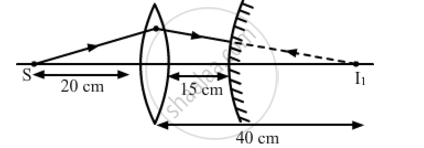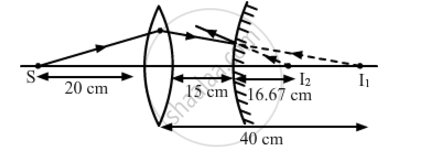# A Convex Lens of Focal Length 20 cm is Placed Coaxially with a Convex Mirror of Radius of Curvature 20 cm. the Two Are Kept at 15 Cm Apart. - Physics

A convex lens of focal length 20 cm is placed coaxially with a convex mirror of radius of curvature 20 cm. The two are kept at 15 cm apart. A point object lies 60 cm in front of the convex lens. Draw a ray diagram to show the formation of the image by the combination. Determine the nature and position of the image formed

#### Solution

Let us first locate the image of the point object S formed by the convex lens.

Here:
u = -60 cm

and f = 20 cm

From the lens formula, we have:

1/v-1/u=1/f

=>1/v=1/f+1/u

=>1/v=1/20+1/((-60))

=>1/v=(3-1)/60=2/60

⇒ 30 cm

The positive sign shows that the image is formed to the right of the lens, as shown in the following figure.The image I1 is formed behind the mirror and hence, acts as a virtual source for the mirror. The convex mirror forms the image I2, whose distance from the mirror can be calculated as:

1/v+1/u=1/f

Here:

u = 15 cm

And

f=R/2=10 cm

=>1/v=1/f-1/u

=>1/v=1/10-1/15

=>1/v=(3-2)/30=1/30

⇒ 30 cm

Hence, the final virtual image is formed at a distance of 30 cm from the convex mirror, as shown in the figure below.Concept: Lenses
Is there an error in this question or solution?
2013-2014 (March) All India Set 1

Share